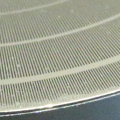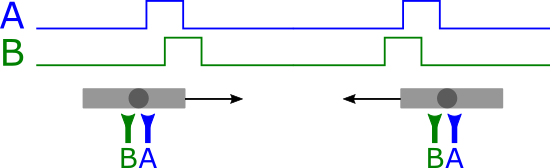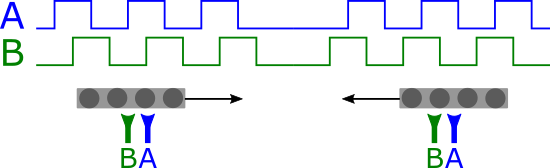Decoding quadrature signals

# Decoding quadrature signalsThe Yocto-PWM-Rx is a sensor with two inputs designed to measure electrical pulse trains. Up to now, we measured the signal on each input for itself, independently from the other input. Now, on top of this, you can analyze the two inputs as a pair of quadrature encoded signals.

A quadrature encoder is a device used to transmit the linear or angular position of a mobile item, using only two digital lines. It is based on the fact that comparing two shifted pulses enables us to determine movement direction:The pulse order on the two sensors indicates movement direction

If we extend this principle by repeating the pattern, we obtain two shifted periodic shifted signals. The shift direction indicates the direction while the pulse count measures the distance:The shift between the two signals indicates movement direction

Thus, if we add:

• +1 each time signal A goes up while signal B is low,
• +1 each time signal B goes up while signal A is high,
• -1 each time signal A goes down while signal B is low, and
• -1 each time signal B goes down while signal A is high,

then we can precisely measure the object position, without being perturbed by possible goings and comings or sensor hesitations on transition zones. We are not talking about absolute positioning, as we are lacking a unique reference for the starting point, but about relative positioning with regards to a given time.

This type of quadrature encoder is used in particular to make angular sensors with a digital output and an accuracy up to the tenth of degree. In this case, there is often a third signal giving a unique pulse at angle zero, thus providing an absolute reference on top of the quadrature incremental coding.

If you update the firmware of your Yocto-PWM-Rx, you can then have access to the quadratureDecoder function. You can enable this function if necessary so that the module automatically decodes the position, if your inputs correspond to a quadrature coded signal. On top of the position, the quadrature decoder automatically computes the movement/rotation speed every 100ms. The speed corresponds to the number of pulses per second, and the sign indicates movement direction.

Here is a short Python example illustrating how to use this new function.

quadrature = YQuadratureDecoder.FirstQuadratureDecoder()
quadrature.set_currentValue(0)

while True:
print("Position: %d " % quadrature.get_currentValue() + \
+ "(Vitesse: %d steps/s)" % quadrature.get_speed() + \
+ " -- Press Ctrl-c to stop")
YAPI.Sleep(1000)

And here is a short demo of an angle sensor using an incremental quadrature encoder output, with a resolution of 0.025 degrees, ie.14'400 positions for a single rotation !

 Add a comment No comment yet Back to blog